Next: Using Additional Information for Up: Update Rules Previous: Avoiding Problems Originating from

### Stabilising the Fixed-Point Update Rules.

The adaptations rules in (28) and (30) assume other parameters to be constant. The weights, sources and biases are updated all at once, however, because it would not be computationally efficient to update only one at a time. The assumption of independence is not necessarily valid, particularly for the posterior means of the variables, which may give rise to instabilities. Several variables can have a similar effect on outputs and when they are all updated to the values which would be optimal given that the others stay constant, the combined effect is too large.

This type of instability can be detected by monitoring the directions of updates of individual parameters. When the problem of correlated effects occurs, consecutive updated values start oscillating. A standard way to dampen these oscillations in fixed point algorithms is to introduce a learning parameterfor each parameter and update it according to the following rule: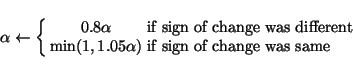(30)

This gives the following fixed point update rules for the posterior means and variances of the sources, weights and the biases: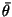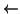(31)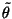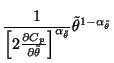(32)

The reason why a weighted geometric rather than arithmetic mean is applied to the posterior variances is that variance is a scale parameter. The relative effect of adding a constant to the variance varies with the magnitude of the variance whereas the relative effect of multiplying by a constant is invariant.Next: Using Additional Information for Up: Update Rules Previous: Avoiding Problems Originating from
Harri Lappalainen
2000-03-03